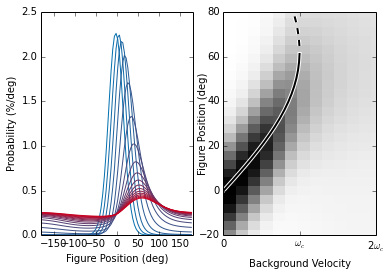# Asymmetric processing of visual motion for simultaneous object and background responses

Fenk LM*, Poehlmann A*, Straw AD (* equal contribution) (2014) Asymmetric processing of visual motion for simultaneous object and background responses. Current Biology. doi:10.1016/j.cub.2014.10.042

## View the models

You can view the models:
The phenomenological model was written such that it can be viewed as a webpage. This is shown below. The physiological model, which closely follows Lindemann et al., (2006), can be downloaded in its original form with the Supplemental Code S1 with our paper or updated versions can be viewed in our github repository. Detailed instructions of how to run each model are in the README.md files at github.

Without further ado, below are the details of our phenomenological model.

# The phenomenological model

Here we describe a phenomenological model of fly visual behaviors based on a few well-known properties of wide-field motion integrators such as the lobula plate tangential cells (LPTCs) of flies. A primary goal was to formulate a very simple model to facilitate rigorous mathematical analysis. It predicts turning responses of the fly to piecewise-defined open- and closed-loop stimuli. The model abstracts fly behavior to a one degree of freedom system (azimuth) and reduces stimuli to horizontal piecewise-defined patterns at zero elevation. We provide a theoretical framework to analytically predict turning responses here, as well as a software implementation in Supplemental Code S1 (also available at http://strawlab.org/asymmetric-motion/). All retinal positions are specified as azimuthal angle from the visual midline (right handed coordinate system).

In :
import itertools

get_ipython().magic("matplotlib inline")  # required for ipynb compability
import matplotlib.pyplot as plt
import matplotlib.colors
import mpl_toolkits.axes_grid1
import numpy as np
import scipy.integrate
import scipy.interpolate
import sympy
import sympy.abc


The internal parameters of the model are, with a single exception, based exclusively on measurements from electrophysiological experiments of well investigated LPTCs, Drosophila Horizontal System (HS) cells Schnell et al. (2010). The remaining free parameter, $C_\text{T}$, is used to account for experimental coupling conditions and predict behavioral responses under a variety of those conditions. The parameter is a constant specifying the cell output to torque coupling, and we discuss it below.

## The motion response

A fundamental property of an HS-cell is its change in membrane potential when a wide-field stimulus, such as horizontal sinusoidal grating covering the whole visual field, is rotated azimuthally around the fly. We call the function that describes the dependence of membrane potential responses to movement of the visual stimulus the motion response. Its dependent variable is the temporal frequency, $f_{\text{t}}$, which is described by $f_{\text{t}} = \omega / \lambda_{\text{p}}$ given a stimulus with a pattern wavelength, $\lambda_{\text{p}}$, and an angular velocity, $\omega$. We use the measured relative motion response from Fig. 2B of Schnell et al. (2010), to fit the parameters of our function. The mathematical form of our function is given by the Reichardt correlator as in Borst, Bahde (1986) after Reichardt, Varju (1959). Like most LPTCs, HS-cells respond with depolarizing and hyperpolarizing responses in the so-called preferred and null directions, respectively. The relative motion response to preferred direction stimuli with constant pattern wavelength is:

Adjusting the parameter, $f_{\text{opt}} = \tau_{\text{opt}}^{-1}$, to $1\,\mathrm{Hz}$, approximates the data reasonably well (Supplemental Fig. S2A).

In :
fig = plt.figure()
fig.suptitle("Preferred Direction Motion Response")

# Motion response data from Schnell et al. 2010 Figure 2B
SCHNELL_MR2BA = np.array([[0.113636, 0.541573],
[0.520833, 0.676404],
[0.757575, 0.721348],
[1.022727, 0.885393],
[1.212121, 0.883146],
[1.505681, 0.795505],
[1.998106, 0.730337],
[3.011363, 0.528089],
[5.009469, 0.337078]]).T
A_wavelength = 22
MR2BA_tf, MR2BA = SCHNELL_MR2BA
MR2BA_av, MR2BA_n = MR2BA_tf * A_wavelength, MR2BA / MR2BA.max()

SCHNELL_MR2BB = np.array([[0.104166, 0.447191],
[0.511363, 0.768539],
[0.748106, 0.764044],
[1.013257, 0.883146],
[1.193181, 0.802247],
[1.496212, 0.782022],
[2.007575, 0.678651],
[3.020833, 0.476404],
[5.018939, 0.377528]]).T
B_wavelength = 45
MR2BB_tf, MR2BB = SCHNELL_MR2BB
MR2BB_av, MR2BB_n = MR2BB_tf * B_wavelength, MR2BB / MR2BB.max()

# Plot the experimental data against angular velocity
ax_omega = plt.subplot2grid((1, 2), (0, 0))
ax_omega.set_xlabel("Angular Velocity (deg/s)")
ax_omega.set_ylabel("Response (a.u.)")
ax_omega.set_xlim(0, 250.)
ax_omega.set_ylim(0, 1.1)
ax_omega.plot(MR2BA_av, MR2BA_n, color="black", marker="s", lw=2, markersize=3.)
ax_omega.plot(MR2BB_av, MR2BB_n, color="grey", marker="o", lw=2, markersize=3.)

# Preferred direction motion response approximation
def MotionResponsePD(temporal_frequency, optimal_frequency):
"""motion response in preferred direction"""
_tf = temporal_frequency / optimal_frequency
return 2 * _tf / (1 + _tf**2)  # the factor 2 normalizes the reponse

MR_PARAMETERS = {"optimal_frequency": 1.0}

# Experimental data and model response against temporal frequency
ax_tfreq = plt.subplot2grid((1, 2), (0, 1))
ax_tfreq.set_xlabel("Temporal Frequency (Hz)")
ax_tfreq.set_xlim(0, 5.)
ax_tfreq.set_ylim(0, 1.1)
ax_tfreq.set_yticklabels([])
ax_tfreq.plot(MR2BA_tf, MR2BA_n, color="black", marker="s", lw=2, markersize=3.)
ax_tfreq.plot(MR2BB_tf, MR2BB_n, color="grey", marker="o", lw=2, markersize=3.)

# the analytical motion response
tf = np.linspace(0, 5)
ax_tfreq.plot(tf, MotionResponsePD(tf, **MR_PARAMETERS), color="red", lw=2)

Out:
[<matplotlib.lines.Line2D at 0x2b8371071cd0>]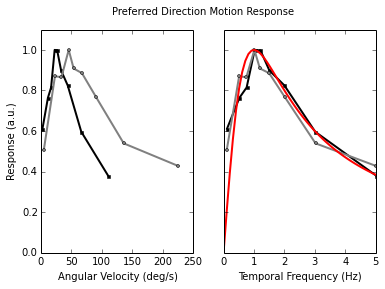A critical feature of our model is the asymmetry of the response to the direction of motion of the stimulus. In particular, the motion response to null direction stimuli is not only inverted in sign but also reduced in amplitude. Basing our estimate on the values published in Fig. 1D of Schnell et al. (2010), we account for this by a factor $C_{\text{ND}} = 0.4$. The relative motion response for stimuli moving in both directions can be written as:

$M(f_{\text{t}}) = \left\{ \begin{array}{rl} M_{\text{PD}}(f_{\text{t}}) &\mbox{ if f_{\text{t}} \ge 0} \\ -C_{\text{ND}} M_{\text{PD}}(-f_{\text{t}}) &\mbox{ otherwise} \end{array} \right.$

The normalized response to a moving sinusoidal pattern in both the null (amplitude is negative) and the preferred (amplitude is positive) direction in temporal frequency space, $f_{\text{t}} = \omega/\lambda_{\text{p}}$, for a pair of neurons with opposite direction selectivities (e.g. contralateral cells) is shown in Supplemental Fig. S2B.

In :
#plt.figure(figsize=(10, 4.5))
plt.figure()
plt.title("Asymmetric Motion Response")
plt.xlabel("Temporal Frequency (Hz)")
plt.ylabel("Response (a.u.)")
plt.xlim(-5., 5.)
plt.ylim(-0.5, 1.1)

class motion_response_pattern_wavelength(object):
def __init__(self, optimal_frequency, asymmetry,
pattern_wavelength, preferred_direction):
assert preferred_direction in ["CW", "CCW"]
if preferred_direction == "CW":
self._sign = 1
else:
self._sign = -1
self._fopt = optimal_frequency
self._asym = asymmetry
self._lambdap = pattern_wavelength

def _mrpd(self, tf):
return MotionResponsePD(tf, self._fopt)

def __call__(self, omega):
tf = self._sign * np.atleast_1d(omega) / self._lambdap
sign = np.clip(np.sign(tf), -self._asym, 1)
return sign * self._mrpd(np.abs(tf))

# Constant taken from Fig 1D
MR_PARAMETERS["asymmetry"] = 0.4
# Motion responses of both cells:
test_pattern_wavelength = np.pi / 4.

mr_l = motion_response_pattern_wavelength(preferred_direction="CW",
pattern_wavelength=test_pattern_wavelength, **MR_PARAMETERS)
mr_r = motion_response_pattern_wavelength(preferred_direction="CCW",
pattern_wavelength=test_pattern_wavelength, **MR_PARAMETERS)

# Plot motion response
omega = np.linspace(-5, 5, 1001)
plt.plot(omega, mr_l(omega), "r", lw=2)  # left cell
plt.plot(omega, mr_r(omega), "b", lw=2)  # right cell

Out:
[<matplotlib.lines.Line2D at 0x2b8371213910>]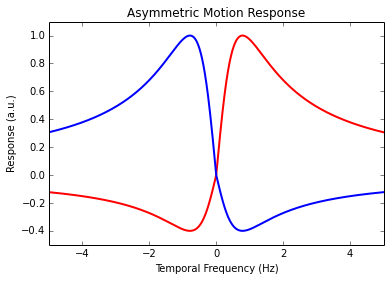## The receptive field

For a small stimulus, the response of an LPTC is also dependent on retinal position. We use the local motion sensitivity measured at zero degrees elevation from Fig. 3C of Schnell et al. (2010) to define the spatial receptive field for our model. Because we simulated visual stimuli along the unit circle, the receptive field is required to be $2\pi$ periodic, and we therefore choose a trigonometric function. However, its exact nature is not important and was chosen for convenience. Our model receptive field (see Supplemental Fig. S2C) is given as:

In this model, $\varphi_{\text{max}}$ is the azimuthal angle at which the model cell responds most strongly to a small field stimulus and the factor $\frac{8}{5\pi}$ normalizes the area under the curve to 1.

In :
plt.figure()
plt.title("Receptive Field")
plt.xlabel("Angular Position (deg)")
plt.ylabel("Response (a.u.)")
plt.xlim(-180., 180.)
plt.xticks([-180, -120, -60, 0, 60, 120, 180])
plt.ylim(0., 0.6)
plt.yticks([8 / (5 * np.pi)], [u"8/5\u03C0"])

# Receptive Field from Fig 3C
SCHNELL_RF3C2Y = np.array([0.12, 0.32, 0.38, 0.35, 0.28, 0.32, 0.33, 0.38,
0.40, 0.44, 0.50, 0.63, 0.72, 0.76, 0.83, 0.84,
0.87, 0.95, 0.95, 0.92, 0.90, 0.90, 0.86, 0.83,
0.72])
SCHNELL_RF3C2X = np.linspace(-42, 94, 25)

RF_X = SCHNELL_RF3C2X
RF_Y = SCHNELL_RF3C2Y / SCHNELL_RF3C2Y.max() * 8 / (5 * np.pi)

phi = np.linspace(-np.pi, np.pi, 101)

def receptive_field(phi, phi_max):
return 8 / (5 * np.pi) * np.cos((phi - phi_max) / 2.) ** 6

# Plot experimental data
plt.plot(RF_X, RF_Y, color="black", marker="s", ls="", markersize=3.)
plt.plot(np.degrees(phi), receptive_field(phi, **RF_PARAMETERS), "r-", lw=2)

Out:
[<matplotlib.lines.Line2D at 0x2b83712138d0>]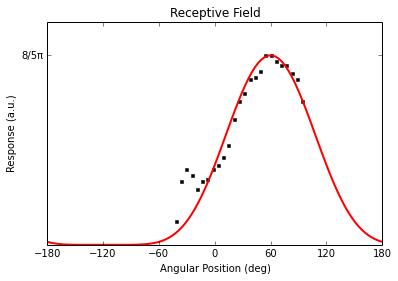The receptive fields of the left (red) and right (blue) model cells are symmetric around zero, as shown in Supplemental Fig. S2D.

In :
plt.figure()
plt.title("Receptive Fields")
plt.xlabel("Angular Position (deg)")
plt.ylabel("Response (a.u.)")
plt.xlim(-180., 180.)
plt.xticks([-180, -120, -60, 0, 60, 120, 180])
plt.ylim(0., 0.6)
plt.yticks([8 / (5 * np.pi)], [u"8/5\u03C0"])
# left cell
plt.plot(np.degrees(phi), receptive_field(phi, **RF_PARAMETERS), "r-", lw=2)
# right cell
plt.plot(-np.degrees(phi), receptive_field(phi, **RF_PARAMETERS), "b-", lw=2)

Out:
[<matplotlib.lines.Line2D at 0x2b83711a8510>]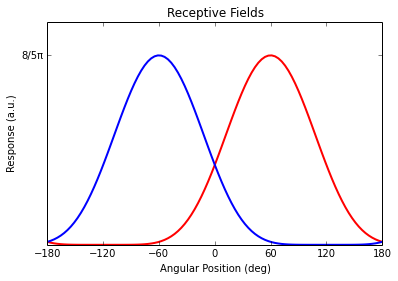## The cell's response

We model the cell's complete output as the integral over all angular positions weighted with the receptive field:

For clarity, we expand this to explicitly show all parameters (enclosed in square brackets):

$W(f_{\text{t}}, [\varphi_{\text{max}}, f_{\text{opt}}, C_{\text{ND}}]) = \int_{-\pi}^{\pi} R(\varphi, [\varphi_{\text{max}}]) M(f_{\text{t}},[ f_{\text{opt}}, C_{\text{ND}}]) \,\mathrm{d}\varphi$

As described above, each of these three parameters, $\varphi_{\text{max}}, f_{\text{opt}}, C_{\text{ND}}$, was adjusted by eye to the experimental data of Schnell et al. (2010). This model describes the noise-free normalized graded potential of a wide-field motion integrator cell. We do not adjust these parameters for any predictions that we make later.

## Introducing the figure-ground stimulus

So far, the model did not consider responses to spatially complex stimuli. The motion response (equation 1) was defined for a panoramic sinusoidal grating, which for a certain angular velocity, $\omega$, and pattern wavelength, $\lambda_{\text{p}}$, generates a temporal frequency, $f_{\text{t}}=\omega / \lambda_{\text{p}}$. The receptive field (equation 2) was defined for a small stimulus. We now introduce a figure-ground stimulus, where the background pattern is occluded by the figure, with the following parameters:

• figure width $\beta$
• figure position $\varphi_{\text{F}}$
• figure pattern wavelength ${\lambda_{\text{F}}}$
• figure velocity $\omega_{\text{F}}$
• ground pattern wavelength $\lambda_{\text{G}}$
• ground velocity $\omega_{\text{G}}$

All the parameters above are inherent to the stimulus, not to the model. This is important because the responses to the figure or to the background --- which we derive below --- evolve from these parameters, and are not properties of the model cell. Please note that in this text, we use the word "figure" to describe a small-field visual object, even when there is no background contrast.

To use these stimulus parameters in the model, we define $\omega_{\text{FG}}$ and $\lambda_{\text{FG}}$ dependent on the angular position, $\varphi$, the figure position, $\varphi_{\text{F}}$, and width, $\beta$, for the figure-ground stimulus.

$\lambda_{\text{FG}}(\varphi, [\varphi_{\text{F}}, \beta]) = \left\{ \begin{array}{rl} \lambda_{\text{F}} & \mbox{ if \varphi_{\text{F}}-\frac{\beta}{2} \le \varphi \le \varphi_{\text{F}}+\frac{\beta}{2} } \\ \lambda_{\text{G}} & \mbox{ otherwise } \\ \end{array} \right.$

$\omega_{\text{FG}}(\varphi, [\varphi_{\text{F}}, \beta]) = \left\{ \begin{array}{rl} \omega_{\text{F}} & \mbox{ if \varphi_{\text{F}}-\frac{\beta}{2} \le \varphi \le \varphi_{\text{F}}+\frac{\beta}{2} } \\ \omega_{\text{G}} & \mbox{ otherwise } \\ \end{array} \right.$

With this we can rewrite the cell response as:

$\begin{split} W(f_{\text{t}}) &= W(\omega_{\text{FG}} / \lambda_{\text{FG}}) = \int_{-\pi}^{\pi} R(\varphi) M(\omega_{\text{FG}} / \lambda_{\text{FG}})\,\mathrm{d}\varphi \\ &= \int_{-\frac{1}{2}\beta}^{+\frac{1}{2}\beta} R(\varphi_{\text{F}} + \varphi)M(\omega_{\text{F}} / \lambda_{\text{F}})\,\mathrm{d}\varphi + \int_{+\frac{1}{2}\beta}^{2\pi-\frac{1}{2}\beta} R(\varphi_{\text{F}} + \varphi) M(\omega_{\text{G}} / \lambda_{\text{G}})\, \mathrm{d}\varphi \end{split}$

We can interpret these two summands as a response to the figure, and a response to the background. But, again, they originate from the properties of the stimulus, not from the properties of the wide-field motion integrator. We define the figure and ground responses as:

$W_{\text{F}}(\varphi_{\text{F}}, \omega_{\text{F}}, [\lambda_{\text{F}}, \beta]) = \int_{-\frac{1}{2}\beta}^{+\frac{1}{2}\beta} R(\varphi_{\text{F}} + \varphi)M(\omega_{\text{F}} / \lambda_{\text{F}})\,\mathrm{d}\varphi$

$W_{\text{G}}(\varphi_{\text{F}}, \omega_{\text{G}}, [\lambda_{\text{G}}, \beta]) = \int_{+\frac{1}{2}\beta}^{2\pi-\frac{1}{2}\beta} R(\varphi_{\text{F}} + \varphi) M(\omega_{\text{G}} / \lambda_{\text{G}})\, \mathrm{d}\varphi$

Since the basic property of our figure-ground stimulus is that the figure occludes the background, the background response, $W_{\text{G}}$, is of course dependent on the figure position, $\varphi_{\text{F}}$, as a variable and the figure width, $\beta$, as a parameter.

If we use a figure of width, $\beta$, and pattern wavelength, $\lambda_{\text{F}}$, in front of a contrast-free background ($\lambda_{\text{G}} = \infty$), we can plot the response of the cell in phase space (see Supplemental Fig. S2F).

In :
plt.figure()

class receptive_field_figure_width(object):
def __init__(self, figure_width, phi_max, position):
assert 0 <= figure_width <= 2 * np.pi
assert -np.pi <= phi_max <= np.pi
assert position in ["left", "right"]
self._fwhalf = figure_width / 2.
self._phi_max = abs(phi_max) if position is "left" else -abs(phi_max)
_root = sympy.integrate(
8 / (5 * sympy.pi) * sympy.cos(sympy.abc.x / 2) ** 6,
sympy.abc.x)
self._rootfunc = sympy.lambdify(sympy.abc.x, _root, np)

def __call__(self, phi, background=False):
phi = phi - self._phi_max
if not background:
return (self._rootfunc(phi + self._fwhalf)
- self._rootfunc(phi - self._fwhalf))
else:
return (self._rootfunc(phi - self._fwhalf + 2 * np.pi)
- self._rootfunc(phi + self._fwhalf))

class cell_response(object):
def __init__(self, position, figure_width,
pattern_wavelength_fg, pattern_wavelength_bg=np.inf):
assert position in ["left", "right"]
if position == "left":
PD = "CW"
else:
PD = "CCW"
self._mrf = motion_response_pattern_wavelength(preferred_direction=PD,
pattern_wavelength=pattern_wavelength_fg, **MR_PARAMETERS)
self._mrg = motion_response_pattern_wavelength(preferred_direction=PD,
pattern_wavelength=pattern_wavelength_bg, **MR_PARAMETERS)
self._rf = receptive_field_figure_width(position=position,
figure_width=figure_width, **RF_PARAMETERS)

def __call__(self, phi, omega_F, omega_G=0):
return (self._rf(phi) * self._mrf(omega_F) +
self._rf(phi, background=True) * self._mrg(omega_G))

class torque_response(object):
def __init__(self, figure_width, pattern_wavelength_fg,
pattern_wavelength_bg=np.inf, ct=1.0):
self._hsl = cell_response("left", figure_width=figure_width,
pattern_wavelength_fg=pattern_wavelength_fg,
pattern_wavelength_bg=pattern_wavelength_bg)
self._hsr = cell_response("right", figure_width=figure_width,
pattern_wavelength_fg=pattern_wavelength_fg,
pattern_wavelength_bg=pattern_wavelength_bg)
self._ct = ct

def __call__(self, phi, omega_F, omega_G=0):
return self._ct * (self._hsl(phi, omega_F, omega_G)
- self._hsr(phi, omega_F, omega_G))

phi, tf_sf = np.meshgrid(np.linspace(-np.pi, np.pi, 101),
extents1f = [-180, 180, -6, 6]

response = torque(phi, tf_sf)

plt.title("Predicted Small-Field Behavioural Response")
plt.xlabel("Angular Position (deg)")
plt.ylabel("Temporal Frequency (Hz)")
im = plt.imshow(response, origin="lower", cmap=plt.get_cmap("RdBu_r"),
extent=extents1f, aspect='auto')
plt.colorbar(label="Response (a.u.)")

Out:
<matplotlib.colorbar.Colorbar instance at 0x2b83714f0ef0>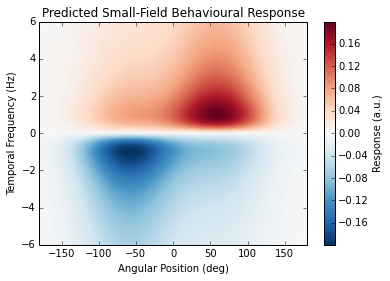## From cell output to torque

We model the torque output of the fly to be proportional to the difference of the left and the right model cell responses. We introduce a factor, $C_{\text{T}}$, which scales the normalized output to torque. This is the only free parameter of our model.

$T(f_{\text{t}}) = C_{\text{T}} \Big[ W^{\text{(left)}}(f_{\text{t}}) - W^{\text{(right)}}(f_{\text{t}}) \Big]$

To simulate a noisy cell response, we add noise to the torque response of our system at this point to get:

$T^{*}(f_{\text{t}}, t) = T(f_{\text{t}}) + N(t) = C_{\text{T}} \Big[ W^{\text{(left)}}(f_{\text{t}}) - W^{\text{(right)}}(f_{\text{t}}) \Big] + N(t)$

We rewrite this torque response for a figure-ground stimulus, using the following abbreviations:

$\begin{split} R_{\text{F}}^{(\text{left/right})}(\varphi_{\text{F}}) &= \int_{-\frac{1}{2}\beta}^{+\frac{1}{2}\beta} R^{(\text{left/right})}(\varphi_{\text{F}} + \varphi)\,\mathrm{d}\varphi \\ R_{\text{G}}^{(\text{left/right})}(\varphi_{\text{F}}) &= \int_{+\frac{1}{2}\beta}^{2\pi - \frac{1}{2}\beta} R^{(\text{left/right})}(\varphi_{\text{F}} + \varphi)\,\mathrm{d}\varphi \\ X^{(-)} &= X^{\text{(left)}} - X^{\text{(right)}} \\ X^{(+)} &= X^{\text{(left)}} + X^{\text{(right)}} \end{split}$

Putting this and the figure-ground stimulus parameters into $T(f_{\text{t}})$ gives:

$\begin{split} T(f_{\text{t}}) &= T(\varphi_{\text{F}}, \omega_{\text{F}}, \omega_{\text{G}}, [\lambda_{\text{F}}, \lambda_{\text{G}}, \beta]) = C_{\text{T}} W^{(-)}(\varphi_{\text{F}}, \omega_{\text{F}}, \omega_{\text{G}}, [\lambda_{\text{F}}, \lambda_{\text{G}}, \beta]) \\ &= C_{\text{T}} \Big( W^{(-)}_{\text{F}}(\varphi_{\text{F}}, \omega_{\text{F}}, [\lambda_{\text{F}}, \beta]) + W^{(-)}_{\text{G}}(\varphi_{\text{F}}, \omega_{\text{G}}, [\lambda_{\text{G}}, \beta]) \Big) \\ \end{split}$

For the sake of simplicity, we set the background contrast to zero for now ($\lambda_{\text{G}} = \infty \rightarrow W_{\text{G}}^{(-)} = 0$).

$T(\varphi_{\text{F}}, \omega_{\text{F}}) = \frac{C_{\text{T}}}{2} \Big[ R_{\text{F}}^{(-)}(\varphi_{\text{F}}) M^{(+)}(\omega_{\text{F}} / \lambda_{\text{F}}) + R_{\text{F}}^{(+)}(\varphi_{\text{F}}) M^{(-)}(\omega_{\text{F}} / \lambda_{\text{F}}) \Big]$

This gives the response properties of a figure in front of a homogeneous background. For different figure widths, the phase space representation of this output is shown in Supplemental Fig. S2E. The receptive fields of both model cells make the initially homogeneous and optomotor like torque response ($360\,^{\circ}$) more and more position specific with decreasing figure width.

In :
fig = plt.figure()
grid = mpl_toolkits.axes_grid1.ImageGrid(fig, 111, (5, 2), axes_pad=0.1)

phi, omega = np.meshgrid(np.linspace(-np.pi, np.pi, 101),
np.linspace(-np.pi, np.pi, 101))

widths = np.radians([360, 270, 180, 90, 45])
positions = ["left", "right"]

griddata = {}
for i, (w, p) in enumerate(itertools.product(widths, positions)):
HS = cell_response(p, figure_width=w, pattern_wavelength_fg=lambdap)
DATA = HS(phi, omega)
griddata[i] = DATA

VMAX, VMIN = 1, -1

for i, data in griddata.items():
grid[i].imshow(data, origin="lower", vmin=VMIN, vmax=VMAX,
cmap=plt.get_cmap("RdBu_r"))
grid[i].set_xticks([1, 51, 101])
grid[i].set_xticklabels([-180, 0, 180 if i == 9 else ""])
grid[i].set_yticks([1, 51, 101])
grid[i].set_yticklabels([-8, 0, 8 if i < 2 else ""])

grid.set_ylabel("Temporal Frequency (Hz)")
grid.set_xlabel("Angular Position (deg)", x=1.)
grid.set_title("left\ncell")
grid.set_title("right\ncell")
grid.text(115, 110, "figure\nwidth")
grid.text(110, 40, "360 deg")
grid.text(110, 40, "270 deg")
grid.text(110, 40, "180 deg")
grid.text(110, 40, "90 deg")
grid.text(110, 40, "45 deg")

Out:
<matplotlib.text.Text at 0x2b837c937a90>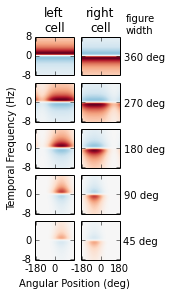Note: The mathematical conversion on the figure terms can be applied to the ground terms in the same manner. For the figure-ground stimulus with $\lambda_{\text{G}} < \infty$ we would get:

$\begin{split} T(\varphi_{\text{F}}, \omega_{\text{F}}, \omega_{\text{G}}) &= \frac{C_{\text{T}}}{2} \Big[ R_{\text{F}}^{(-)}(\varphi_{\text{F}}) M^{(+)}(\omega_{\text{F}} / \lambda_{\text{F}}) + R_{\text{F}}^{(+)}(\varphi_{\text{F}}) M^{(-)}(\omega_{\text{F}} / \lambda_{\text{F}}) \Big] \\ &+ \frac{C_{\text{T}}}{2} \Big[ R_{\text{G}}^{(-)}(\varphi_{\text{F}}) M^{(+)}(\omega_{\text{G}} / \lambda_{\text{G}}) + R_{\text{G}}^{(+)}(\varphi_{\text{F}}) M^{(-)}(\omega_{\text{G}} / \lambda_{\text{G}}) \Big] \end{split}$

## The dynamic equation

Above we derived an analytical model for the torque output of a fly based on the fundamental properties of motion integrator cells. In the following sections, we use this model to predict fly behavior during different figure-ground discrimination tasks, where two coupling conditions will be important. These are closed-loop and open-loop. For an open-loop stimulus, the torque produced does not influence the stimulus. The stimulus moves along a predefined trajectory in space time, and we measure torque output over time. In a closed-loop scenario, we apply the torque produced by the model on the part of the stimulus in closed-loop. For example, for a closed-loop figure (position: $\varphi_{\text{F}}$, velocity: $\dot{\varphi}_{\text{F}}$) with no or stationary background, we obtain a Langevin type dynamic equation of the form:

$\begin{split} \Theta \ddot{\varphi}_{\text{F}} &= T^{*}(\varphi_{\text{F}}, \dot{\varphi}_{\text{F}}) \\ &= \frac{C_{\text{T}}}{2} \Big[ R_{\text{F}}^{(-)}(\varphi_{\text{F}}) M^{(+)}(\dot{\varphi}_{\text{F}} / \lambda_{\text{F}}) + R_{\text{F}}^{(+)}(\varphi_{\text{F}}) M^{(-)}(\dot{\varphi}_{\text{F}} / \lambda_{\text{F}}) \Big] + N(t) \end{split}$

Here we introduced an additional constant, $\Theta$, which describes the effective moment of inertia in the model. In our tethered flight experiments, this corresponds to the inverse of the coupling gain, $g$, described in the manuscript. As can be seen easily $\Theta$ and $C_\text{T}$ are not linearly independent, so for this case only the sign of $\Theta$ will be important. The case where $\Theta$ is set to a negative value corresponds to the natural condition in which the generated torque moves the figure in the opposing direction, referred to as normal gain.

## Equivalence with Poggio and Reichardt 1973 model for specific conditions

As mentioned in the main text, because this analytical model is based explicitly on a pair of visual neurons, it can predict responses to arbitrary visual stimuli in both open- and closed-loop. We found that for a particular stimulus configuration (closed-loop figure fixation without input from a visual background), it is formally equivalent to the classical model proposed by Poggio and Reichardt based on torque measurements Poggio, Reichardt (1973), Reichardt, Poggio (1975) (Eq. 2). For the specific notation refer to Reichardt, Poggio (1975). One can identify $\begin{split} R_{\text{F}}^{(-)}(\varphi_{\text{F}}) M^{(+)}(\dot{\varphi}_{\text{F}} / \lambda_{\text{F}})\; &\widehat{=} \; D^{\dagger}(\varphi(t), \dot{\varphi}(t)) \\ R_{\text{F}}^{(+)}(\varphi_{\text{F}}) M^{(-)}(\dot{\varphi}_{\text{F}} / \lambda_{\text{F}})\; &\widehat{=} \;\rho(\varphi(t), \dot{\varphi}(t)) \end{split}$ as the even ($f(x)=f(-x)$) and odd ($f(x)=-f(-x)$) symmetric parts of the torque response in $\dot{\varphi}$.

We now linearize the Langevin equation around $\dot{\varphi}_{\text{F}} = 0$. Since our system is described by stochastic processes we use Kazakov (1961) to justify the linearization. This assumes that the temporal average of the squared velocity does not vanish $\langle\dot{\varphi}^2\rangle > 0$ and the figure is almost stationary $\dot{\varphi} \approx 0$, which is a valid assumption since we are only interested in the quasi stationary behavior of the system.

For the even term, the linearization around $\dot{\varphi} \approx 0$ returns a constant and terms of the order of 2 or higher. $\langle M^{(+)}(\dot{\varphi}) \rangle = M^{(+)}_0 + O(\dot{\varphi}^2)$ which depends on the initial slope of the motion response and on the asymmetry, $C_{\text{ND}}$, of the motion responses. For a symmetric motion response, this constant would be equal to zero. The asymmetry is therefore required for the system to have a restoring force, which is essential for figure fixation. Additionally, it is important to understand that $M^{(+)}_0 = 0$ if $\langle\dot{\varphi}^2\rangle = 0$, i.e. in the absence of noise. It is a common misunderstanding that the linearization would throw away the asymmetric response to a moving figure.

After linearizing the even term, we can write: $\frac{C_{\text{T}}}{2} R_{\text{F}}^{(-)}(\varphi_{\text{F}}) M^{(+)}_0 = \frac{\partial}{\partial \varphi} U(\varphi)$ Which describes the restoring force of the system. Since we assume the potential energy of the dynamic system to be path independent, we can define a scalar potential, $U(\varphi)$, as written above. Due to our circular boundary conditions, $U(\varphi) = U(\varphi + n 2\pi)$ is the periodic potential in which the figure is moving. Please note that this potential depends on the shape of the receptive field and, more importantly, on the properties of the presented stimulus. It results from the stimulus properties and a spatially integrating (wide-field) motion responsive cell having an anisotropic receptive field and an asymmetric motion response.

For the odd term, the linearization around $\dot{\varphi} \approx 0$ returns only a linear term (see Kazakov (1961)) and terms of the order of 3 or higher. $\langle M^{(-)}(\dot{\varphi}) \rangle = M^{(-)}_0 \dot{\varphi} + O(\dot{\varphi}^3)$ The odd function $M^{(-)}(\dot{\varphi})$ is by definition already point symmetric and shows no asymmetry. For small velocities this linearization is valid.

After linearizing the odd term, we get: $\frac{C_{\text{T}}}{2} R_{\text{F}}^{(+)}(\varphi_{\text{F}}) M^{(-)}_0 \dot{\varphi} \approx r \dot{\varphi}$ in Reichardt, Poggio (1975), they assume $R^{(+)}(\varphi) = r$ to be constant. Since the odd term describes the dampening force of the system for quasi stationary processes, this assumption is completely reasonable from a modelling perspective (note that $R_{\text{F}}^{(+)}(\varphi) > 0$ is true for all $\varphi$).

With all this, we can write down the linearized torque response to a figure as the Langevin equation:

$\begin{split} \Theta \ddot{\varphi}_{\text{F}} &= T^{*}(\varphi_{\text{F}}, \dot{\varphi}_{\text{F}}) \\ &= \frac{C_{\text{T}}}{2} \Big[ R_{\text{F}}^{(-)}(\varphi_{\text{F}}) M^{(+)}(\dot{\varphi}_{\text{F}} / \lambda_{\text{F}}) + R_{\text{F}}^{(+)}(\varphi_{\text{F}}) M^{(-)}(\dot{\varphi}_{\text{F}} / \lambda_{\text{F}}) \Big] + N(t) \\ &\approx \frac{\partial}{\partial \varphi_{\text{F}}} U(\varphi_{\text{F}}) + r \dot{\varphi}_{\text{F}} + N(t) \end{split}$

using standard techniques, this Langevin equation can be transformed into a Fokker-Planck type equation, which describes the distribution arising from the time evolution of this stochastic differential equation. This representation allowed us to calculate probability distributions for the figure fixation process.

$\frac{\partial}{\partial t} P = -\omega \frac{\partial}{\partial \varphi} P - \frac{\partial}{\partial \omega} \Big(\frac{\partial}{\partial \varphi} U + r \omega \Big) P + \zeta \frac{\partial^2}{\partial \omega^2} P$

For this case, closed-loop figure fixation, this equation is equivalent to the theoretical framework presented in Poggio, Reichardt (1973), Reichardt, Poggio (1975). Again, note that we did not derive this equation from observed torque responses of tethered flies, but from the measured response properties of the wide-field motion integrator cells --- the HS-cells --- in Drosophila. Furthermore, our spatially explicit representation of visual stimuli and our explicit treatment of open- or closed-loop dynamics allow use of our framework in other scenarios, as performed below.

To summarize the above, the subtracted output of two opposing HS-like model cells predicts figure fixation in Drosophila.

## Theoretical predictions

In the following experiments, the fly is in closed-loop control of a figure displayed in front of a moving background. It was previously shown that flies fixate a figure in front of a moving background at a position shifted from zero in the direction opposite to the background movement direction, and it was suggested that this results from a summation of a motion independent response and a motion response (Bahl et al., 2013).

However, we now show that our model, with only a single pathway, also predicts figure displacement. The quasistationary dynamic equation for our system looks like:

$\begin{split} \Theta \ddot{\varphi}_{\text{F}} &= T(\varphi_{\text{F}}, \dot{\varphi}_{\text{F}}, \omega_{\text{G}}) \\ &= \frac{C_{\text{T}}}{2} \Big[ R_{\text{F}}^{(-)}(\varphi_{\text{F}}) M^{(+)}(\dot{\varphi}_{\text{F}} / \lambda_{\text{F}}) + R_{\text{F}}^{(+)}(\varphi_{\text{F}}) M^{(-)}(\dot{\varphi}_{\text{F}} / \lambda_{\text{F}}) \Big] \\ &+ \frac{C_{\text{T}}}{2} \Big[ R_{\text{G}}^{(-)}(\varphi_{\text{F}}) M^{(+)}(\omega_{\text{G}} / \lambda_{\text{G}}) + R_{\text{G}}^{(+)}(\varphi_{\text{F}}) M^{(-)}(\omega_{\text{G}} / \lambda_{\text{G}}) \Big] \end{split}$

We would now like to perform a stability analysis of figure fixation. For this, we calculate the points in space for which the torque response vanishes and then check each of these fixed points to determine if it is a stable or an unstable equilibrium.

Since we are only interested in the fixed points of the system, the forces in this equation should vanish, and we can again linearize around a stable figure position and rewrite the dynamic equation to:

$\Theta \ddot{\varphi}_{\text{F}} = \frac{C_{\text{T}}}{2} \Big[ \frac{\partial}{\partial \varphi_{\text{F}}} V(\varphi_{\text{F}}, \omega_{\text{G}}) + r \dot{\varphi}_{\text{F}} \Big]$

with

$\frac{\partial}{\partial \varphi_{\text{F}}} V(\varphi_{\text{F}}, \omega_{\text{G}}) = \frac{\partial}{\partial \varphi_{\text{F}}} U(\varphi_{\text{F}}) + R_{\text{G}}^{(-)}(\varphi_{\text{F}}) M^{(+)}(\omega_{\text{G}} / \lambda_{\text{G}}) + R_{\text{G}}^{(+)}(\varphi_{\text{F}}) M^{(-)}(\omega_{\text{G}} / \lambda_{\text{G}})$

At the fixed points of the system, the force, $\frac{\partial}{\partial \varphi_{\text{F}}} V(\varphi_{\text{F}}, \omega_{\text{G}})$, vanishes. By solving for the positions where torque was zero, we calculated the fixed points as we altered background velocity, with the results shown in Fig. 3A (top) of the main text.

In :
plt.figure()

FIGURE_WIDTH_DEG = 22.5
BACKGROUND_WAVELENGTH_DEG = 22.5
M0 = 0.1

def figure_potential(phi, omega_bg, figure_width, pwlbg, m0):

rfl = receptive_field_figure_width(position="left",
figure_width=figure_width, **RF_PARAMETERS)
rfr = receptive_field_figure_width(position="right",
figure_width=figure_width, **RF_PARAMETERS)
bgl = cell_response("left", figure_width=figure_width,
pattern_wavelength_fg=np.inf,
pattern_wavelength_bg=pwlbg)
bgr = cell_response("right", figure_width=figure_width,
pattern_wavelength_fg=np.inf,
pattern_wavelength_bg=pwlbg)

return ((rfl(phi) - rfr(phi)) * m0 +
(bgl(phi, 0, omega_G=omega_bg) - bgr(phi, 0, omega_G=omega_bg)))

force = lambda phi, rho: -figure_potential(phi, rho,

rho_deg = np.linspace(0, -0.5, 71)  # The upper bound should be > 2*OMEGAC
phi_deg = np.linspace(0, 180, 51)
RHO_DEG, PHI_DEG = np.meshgrid(rho_deg, phi_deg)
extent = (rho_deg, rho_deg[-1], phi_deg[-1], phi_deg)
clim = 0.5 * max(abs(Z.max()), abs(Z.min()))

plt.imshow(Z, aspect='auto', extent=extent,
clim=(-clim, clim), cmap=plt.get_cmap("PiYG"))
im = plt.colorbar(label='Torque (a.u.)')
quadcontour = plt.contour(RHO_DEG, PHI_DEG, Z, colors='k',
linestyles='dashed', linewidths=2, levels=[0.0])

OMEGAC = None
# color stable branches in green
lines = linecollection.get_segments()
for line in lines:
brho, bphi = line.T
OMEGAC = np.degrees(bx.min())
m2 = stability = (force(by - 0.01, bx) - force(by + 0.01, bx)) >= 0
plt.plot(brho[m2], bphi[m2], color='k', ls='-', lw=2)

plt.xlim(rho_deg, 2 * OMEGAC)
plt.xticks([0, OMEGAC, 2 * OMEGAC], ["0", "$\\omega_{c}$", "2$\\omega_{c}$"])
plt.xlabel("Background Velocity")
plt.ylabel("Figure Position (deg)")
plt.ylim(phi_deg, phi_deg[-1])

Out:
(0.0, 180.0)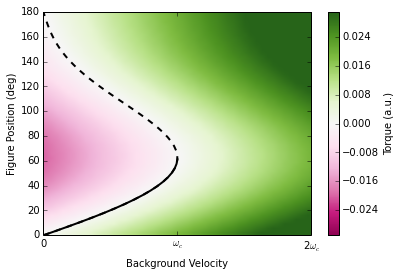We furthermore evaluated whether these were stable or unstable. In Fig. 3A (top), the solid black line is the stable position of a quasistationary figure dependent on the background velocity. At this stable point, the restoring force in the system pushes the figure back to the stable branch (indicated by the color coding). The dashed black line is the unstable position of the figure and perturbation from this equilibrium would grow and push the figure away.

With increasing background velocity, the stable and unstable points approach each other. At a critical background velocity, $\omega_{\text{c}}$, the fixed points meet and vanish (Fig. 3A, top) and therefore the figure can no longer be fixated. This is a classical saddle-node bifurcation. At higher background velocities, the total torque is dominated by the response component due to the open-loop background, much like an optomotor response, and exceeds the fraction generated by the response to the figure.

To calculate the probability distribution, $P(\varphi)$, of figure positions dependent on background velocity, we solve the Fokker-Planck equation derived above, but for the potential, $V(\varphi_{\text{F}}, \omega_{\text{G}})$. The analytical solution for this type of Fokker-Planck equation for arbitrary potentials can be found in Reichardt, Poggio 1975. With this, we can calculate the probability distribution for the figure in closed-loop, and compare it to the stationary solution of the bifurcation (see Supplemental Fig. S2G and Fig. 3A bottom). Note how the mode of the distribution shifts in position and how the distribution becomes flatter when getting closer to the critical velocity.

In :
FIG_WIDTH_RAD = np.radians(FIGURE_WIDTH_DEG)

def NEWpotential(figure_width, m0):
N = 128
rfl = receptive_field_figure_width(position="left",
figure_width=figure_width, **RF_PARAMETERS)
rfr = receptive_field_figure_width(position="right",
figure_width=figure_width, **RF_PARAMETERS)

def _integral_part(phi):
_rf = lambda x: (rfl(x) - rfr(x)) * m0

DATA = []
for pos in np.linspace(0, 2 * np.pi, N, endpoint=False):
DATA.append(_integral_part(pos))
res = np.array(DATA + DATA + DATA)
phi = np.linspace(-2 * np.pi, 4 * np.pi, 3 * N, endpoint=False)
func = scipy.interpolate.interp1d(phi, res)

def _potential(phi):
arg = (phi + np.pi) % (2 * np.pi) - np.pi
return func(arg)

return _potential

def LINpotential(omega_bg, figure_width, pwlbg):

N = 256
bgl = cell_response("left", figure_width=figure_width,
pattern_wavelength_fg=np.inf,
pattern_wavelength_bg=pwlbg)
bgr = cell_response("right", figure_width=figure_width,
pattern_wavelength_fg=np.inf,
pattern_wavelength_bg=pwlbg)

def Vstar(phi):
_cmr = lambda x: -(bgl(x, 0, omega_G=omega_bg)
- bgr(x, 0, omega_G=omega_bg))

DATA = []
phis = np.linspace(-2 * np.pi, 2 * np.pi, N + 1, endpoint=True)
for phi in phis:
DATA.append(Vstar(phi))

res = np.array(DATA)
func = scipy.interpolate.interp1d(phis, res)
return func

func0 = NEWpotential(fw, m0)
func1 = LINpotential(omega_bg, fw, bwl)

def _potential(x):
ret = func1(x) - func0(x)
if np.isscalar(ret):
try:
return ret.asscalar()
except AttributeError:
return ret
else:
return ret

return _potential

def probability(omega_bg, m0, d, c):

N = 91
phis, dphi = np.linspace(0, 2 * np.pi, N, endpoint=False, retstep=True)

eta = d / float(c)
ustar = Ustar(m0, omega_bg)

_f1 = lambda x: np.exp(-eta * ustar(x))

P = []
for phi in phis:
f0 = np.exp(eta * ustar(phi))
f1, _ = scipy.integrate.quad(_f1, phi - 2 * np.pi, phi,
epsabs=1e-3, epsrel=1e-3)
P.append(f0 * f1)

arr = np.array(P)
arr = arr / arr.sum()
return arr, ustar(phis)

plt.figure()
ax0 = plt.subplot2grid((1, 2), (0, 0))
ax1 = plt.subplot2grid((1, 2), (0, 1))

ax0.set_xlabel("Figure Position (deg)")
ax0.set_ylabel("Probability (%/deg)")

DATA = []

LC = matplotlib.colors.LinearSegmentedColormap.from_list(
"FigureGroundPaper",
[(0x05/255., 0x71/255., 0xB0/255., 1.0),
(0xCA/255., 0x00/255., 0x20/255., 1.0)],

PROB, POT = probability(rho, M0, 4., 0.01)
N = len(PROB)/2
PROB = PROB/np.sum(PROB) * len(PROB) / 360. * 100.
ax0.plot(np.linspace(-180, 180, 91, endpoint=False),
np.roll(PROB, N), color=LC(i/M))
DATA.append(np.roll(PROB, N))

ax0.set_ylim(bottom=0)
ax0.set_xlim(-180, 180)

arr = np.vstack(DATA).T[::-1, :]
ax1.imshow(arr,
cmap=plt.get_cmap("Greys"), aspect='auto',

# color stable branches in green
lines = linecollection.get_segments()
for line in lines:
brho, bphi = line.T
m2 = stability = (force(by - 0.01, bx) - force(by + 0.01, bx)) <= 0
ax1.plot(brho[m2], bphi[m2], color='w', ls='--', lw=4)
ax1.plot(brho[~m2], bphi[~m2], color='w', ls='-', lw=4)
ax1.plot(brho[m2], bphi[m2], color='k', ls='--', lw=2)
ax1.plot(brho[~m2], bphi[~m2], color='k', ls='-', lw=2)

ax1.set_ylim(-20, 80)
ax1.set_xlim(rho_deg, 2*OMEGAC)
ax1.set_xticks([0, OMEGAC, 2*OMEGAC])
ax1.set_xticklabels(["0", "$\\omega_{c}$", "2$\\omega_{c}$"])
ax1.set_xlabel("Background Velocity")

<matplotlib.text.Text at 0x2b837cac6610>